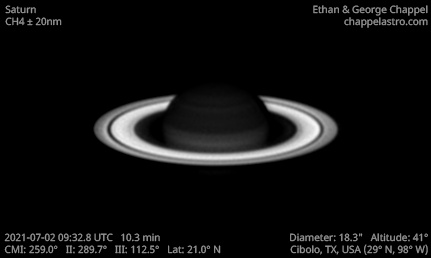# Saturn 2021-07-02 09:32 UTC

CM1: 259.00°

CM2: 289.70°

CM3: 112.50°

CLat: +21.00°

Description

A beautiful night with excellent seeing July 2, 2021. We were prepared this time and took out the EdgeHD 14 to take high resolution images of Saturn.

Many bands are visible across the planet. Despite the high resolution of this image, the north polar hexagon is hard to distinguish other than a slight reddish tinge. In contrast to the yellow-brown and green dominating the northern hemisphere, the southern hemisphere has various shades of blue. When a hemisphere tilts away from the Sun, the rings block most of the sunlight from reaching it. The lack of sunlight causes the ammonia clouds that define Saturn's color to clear out and expose the Hydrogen gas deeper in the atmosphere.

Equipment

ZWO ASI290MM

Celestron EdgeHD 14

Logs
```FireCapture v2.6  Settings
------------------------------------
Observer=Ethan Chappel
Location=Cibolo
Scope=Celestron C14 EdgeHD
Camera=ZWO ASI290MM
Filter=CH4
Profile=Saturn
Diameter=18.23"
Magnitude=0.33
CMI=261.1° CMIII=114.5°  (during mid of capture)
FocalLength=1550mm (F/4)
Resolution=0.39"
Filename=s2021-07-02_09-36-21_ch4_ec.ser
Date=2021_07_02
Start=09_34_51.001
Mid=09_36_21.007
End=09_37_51.013
Start(UT)=09_34_51.001
Mid(UT)=09_36_21.007
End(UT)=09_37_51.013
Duration=180.012s
Date_format=yyyy_MM_dd
Time_format=HH_mm_ss
LT=UT -6h
Frames captured=2572
File type=SER
Binning=no
ROI=320x306
ROI(Offset)=640x24
FPS (avg.)=14
Shutter=70.00ms
Gain=455 (75%)
FPS=100 (off)
AutoHisto=75 (off)
Gamma=50 (off)
USBTraffic=100 (off)
AutoGain=off
HighSpeed=off
AutoExposure=off
SoftwareGain=10 (off)
Brightness=1 (off)
Histogramm(min)=0
Histogramm(max)=135
Histogramm=52%
Noise(avg.deviation)=4.25
Limit=180 Seconds
Sensor temperature=32.3°C
Focuser position=1480
FireCapture v2.6  Settings
------------------------------------
Observer=Ethan Chappel
Location=Cibolo
Scope=Celestron C14 EdgeHD
Camera=ZWO ASI290MM
Filter=CH4
Profile=Saturn
Diameter=18.23"
Magnitude=0.33
CMI=258.9° CMIII=112.4°  (during mid of capture)
FocalLength=1550mm (F/4)
Resolution=0.39"
Filename=s2021-07-02_09-32-42_ch4_ec.ser
Date=2021_07_02
Start=09_31_12.092
Mid=09_32_42.110
End=09_34_12.129
Start(UT)=09_31_12.092
Mid(UT)=09_32_42.110
End(UT)=09_34_12.129
Duration=180.037s
Date_format=yyyy_MM_dd
Time_format=HH_mm_ss
LT=UT -6h
Frames captured=2572
File type=SER
Binning=no
ROI=320x306
ROI(Offset)=640x24
FPS (avg.)=14
Shutter=70.00ms
Gain=455 (75%)
FPS=100 (off)
AutoHisto=75 (off)
Gamma=50 (off)
USBTraffic=100 (off)
AutoGain=off
HighSpeed=off
AutoExposure=off
SoftwareGain=10 (off)
Brightness=1 (off)
Histogramm(min)=0
Histogramm(max)=130
Histogramm=50%
Noise(avg.deviation)=4.06
Limit=180 Seconds
Sensor temperature=32.3°C
Focuser position=1480
FireCapture v2.6  Settings
------------------------------------
Observer=Ethan Chappel
Location=Cibolo
Scope=Celestron C14 EdgeHD
Camera=ZWO ASI290MM
Filter=CH4
Profile=Saturn
Diameter=18.23"
Magnitude=0.33
CMI=257.1° CMIII=110.6°  (during mid of capture)
FocalLength=1550mm (F/4)
Resolution=0.38"
Filename=s2021-07-02_09-29-33_ch4_ec.ser
Date=2021_07_02
Start=09_28_03.569
Mid=09_29_33.591
End=09_31_03.614
Start(UT)=09_28_03.569
Mid(UT)=09_29_33.591
End(UT)=09_31_03.614
Duration=180.045s
Date_format=yyyy_MM_dd
Time_format=HH_mm_ss
LT=UT -6h
Frames captured=2572
File type=SER
Binning=no
ROI=320x306
ROI(Offset)=640x24
FPS (avg.)=14
Shutter=70.00ms
Gain=455 (75%)
FPS=100 (off)
AutoHisto=75 (off)
Gamma=50 (off)
USBTraffic=100 (off)
AutoGain=off
HighSpeed=off
AutoExposure=off
SoftwareGain=10 (off)
Brightness=1 (off)
Histogramm(min)=0
Histogramm(max)=136
Histogramm=53%
Noise(avg.deviation)=4.13
Limit=180 Seconds
Sensor temperature=32.3°C
Focuser position=1480
```